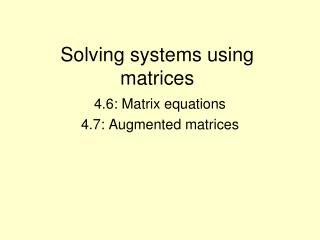DownloadDownload PresentationSolving systems using matrices

# Solving systems using matrices

Télécharger la présentation## Solving systems using matrices

- - - - - - - - - - - - - - - - - - - - - - - - - - - E N D - - - - - - - - - - - - - - - - - - - - - - - - - - -
##### Presentation Transcript

1. Solving systems using matrices 4.6: Matrix equations 4.7: Augmented matrices

2. Solving systems using matrices Matrix equation Augmented matrix constants constants Coefficients Coefficients Variables

3. Matrix Equations • Be sure that the system is lined up before you write matrices • The first matrix is the coefficients of the variables • The second matrix contains the variables (this matrix is only for notation) • The third matrix is the constants (numbers after the equal sign)

4. Solve a matrix equation • Enter the coefficient matrix in as matrix A • Enter the constant matrix in as B • Type in • The solution will show up as a 2x1 matrix EXAMPLE:

5. Examples:

6. Augmented Matrices • Be sure to line up the system first • Put all of the coefficients into one matrix (the # of columns will be 1 more than the # of rows) • In the Matrix Math menu choose option B:rref( • Select matrix A and press enter • The result will be the identity matrix with the solutions to the system in the last column

7. Example:

8. No Solution and Many solutions Matrix equations will result in an error message when the system is no solution OR many solutions Augmented matrices can be identified as no solution or many solutions by looking at the bottom row of the solution

9. The identity matrix will be missing from the solution The system has No Solution if the last row is all zeros with a one at the end of the row The system has Many Solutions if the last row is all zeros

10. Examples: